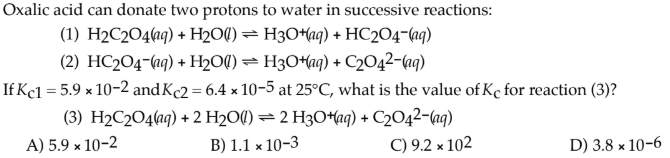# Oxalic acid can donate two protons to water in successive reactions: (1) H2C2O4(aq) + H2O(l) ⇌ H3O+(aq) + HC2O4-(aq) (2) HC2O4-(aq) + H2O(l) ⇌ H3O+(aq) + C2O42-(aq) If Kc1 = 5.9 × 10-2 and Kc2 = 6.4 × 10-5 at 25°C, what is the value of Kc for reaction (3)? (3) H2C2O4(aq) + 2 H2O(l) ⇌ 2 H3O+(aq) + C2O42-(aq) A) 5.9 × 10^-2 B) 1.1 × 10^-3 C) 9.2 × 10^2 D) 3.8 × 10^-6